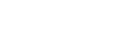# What do we do with Mathjax macro vs Formula macro in XS?

Hi devs,

Now that we have a client-side PDF that properly exports the mathjax macro, we need to decide whether we want to promote the mathjax macro or continue promoting the formula one.

Some considerations:

• The mathjax macro was developed because it produces better rendering than the formula macro
• The formula macro is not very easy to setup since there are several renderers. Also some user is reporting problems about using the formula macro that breaks his wiki in some cases (I don’t have the details).
• With the latest macro discovery feature from Thomas/FASTEN, it’s slightly less important to have macros bundled in XS to be discovered.
• AFAICS the mathjax macro supports more feature than the formula one (including references) and is a superset of it.

I see the following options:

• Option 1: both macros at same level
• Extract the formula macro outside of XS (into xwiki-contrib) and let users decide what extension to install in their wiki.
• We’ll need to recommend one extension or if both have value, then at least do a comparison table and explain when to use one over another
• We probably also need to make the mathjax macro recommended
• Option 2: mathjax macro over formula macro
• Extract the formula macro outside of XS (into xwiki-contrib)
• Add the mathjax macro inside XS in replacement of the formula macro
• Option 3: formula macro FTW
• Deprecate the mathjax macro
• Add a new mathjax “renderer” in the formula macro and make it the default one. Note that when using mathjax you specify the font size in the content and not in the macro parameter as it’s done in the formula macro (but I guess it could be ignored in this case). Similarly mathjax doesn’t produce images so the imageType macro param would also need to be ignored.
• Optional: Also extract the formula macro outside of XS (into xwiki-contrib)

My preference would go to option 1 and recommend the mathjax macro. The rationale for not having it in XS is that I don’t think every user needs a formula macro, it’s a pretty specific case.

WDYT?

Thanks

+1 for Option 1 too

I feel Option 3 is actually pretty much Option 1 but with one more step (make the formula macro support also mathjax) so we can start with Option 1 and see later if someone wants to work on the extra step (but I don’t think it worth it for the same reason people are generally choosing between {{groovy}} and {{python}} and not between {{script language="groovy"}} and {{script language="python"}}).

+1 for option 1 also.

Thanks,
Marius